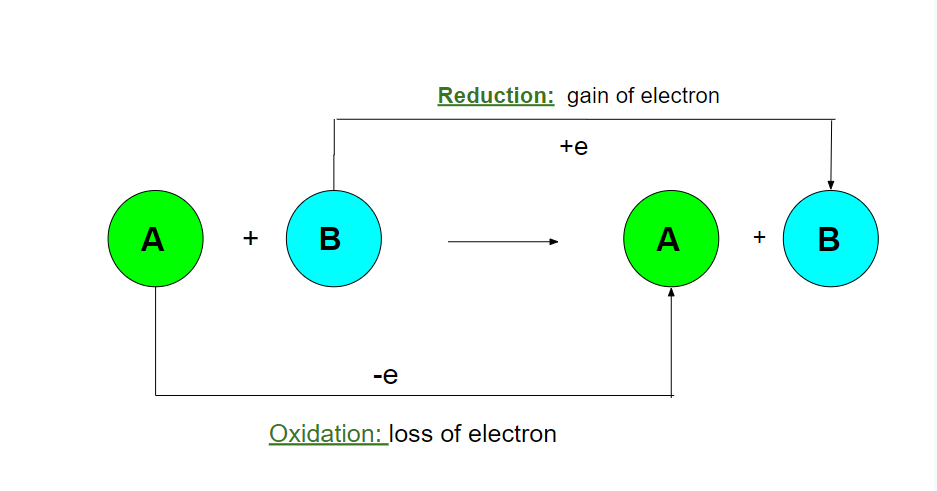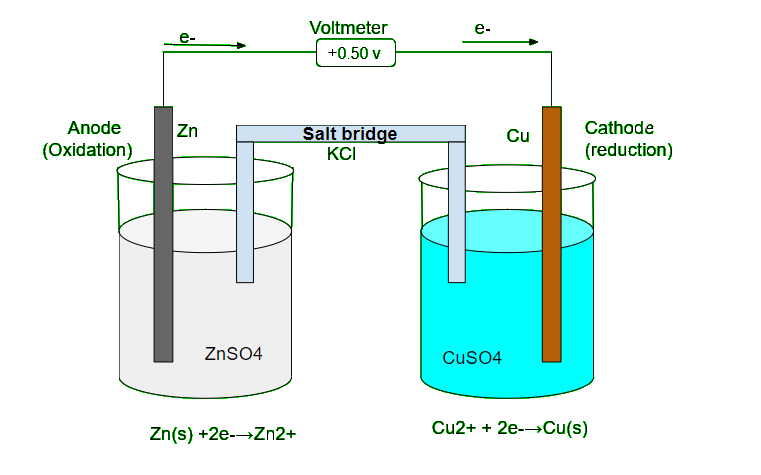GFG App
Open AppBrowser
Continue

# Redox Reactions in terms of Electron Transfer

A variety of chemical and biological reactions like burning of different types of fuels (wood, kerosene, coal, LPG, petrol, diesel), digestion of food in animals, photosynthesis by plants, extraction of aluminum from alumina, electricity generation from batteries or cell, rusting of iron fall in the category of redox reaction.

### What is a Redox Reaction?

Redox reaction is a type of chemical reaction in which oxidation and reduction take place simultaneously. It is the combination of two words that is reduction and oxidation. As shown in the below equation,  here sodium(Na) is oxidized as well as hydrogen(H) is reduced. So, their oxidation, as well as reduction, takes place simultaneously. It involves the transfer of electrons between two species. This reaction is used in pharmaceuticals, biological, industrial, metallurgical and agricultural areas.

For Example:

2Na + H2  → 2NaH### Oxidation and Reduction

• Oxidation: It is defined as the addition of oxygen/electronegative elements to a substance or removal of hydrogen/electropositive elements from a substance.
• For Example:

S (s) + O2 (g)   → SO2 (g)           [oxygen is added]

Mg (s) + Cl2 (g) → MgCl2 (s)     [electronegative element (Cl) is added]

• Reduction: It is defined as the removal of oxygen/electronegative elements from a substance or addition of Hydrogen/electropositive elements to a substance.
• For Example:

CuO (s) + H2 (g)  → Cu (s) + H2O (l)                    [removal of oxygen]

2FeCl3 (aq) + H2 (g)  → 2FeCl2 (aq) + 2HCl      [removal of electronegative elements]

### Redox Reaction on the basis of Electron Transfer

The chemical reaction in which the loss of electrons and gain of electrons take place simultaneously is called a redox reaction.

For Example:

MnO4(aq) + SO32-(aq)  →  MnO42-(aq) + SO42-(aq)       [Mn gained 1 electron and S lost 2 electron]

As shown in the above equation, here magnesium(Mg) gained one electron, as well as Sulphur (S), lost two-electron. So, both oxidation as well reduction takes place simultaneously. This explains that the equation is an example of a redox reaction.

• Oxidation reaction: The loss of electrons by any species in a chemical reaction is known as an oxidation reaction. By losing electrons, the positive charge of the species increases or the negative charge of the species decreases.

For Example,                         2Na   +    Cl2   →   2Na    +    Cl–

Oxidation state:                       0            0             +1           -1

As shown in the above equation, the oxidation state of Sodium(Na) changed from 0 to 1 which means it lost one electron or the positive charge of Sodium(Na) is increased.

• Reduction reaction: The gain of electrons by any species in a chemical reaction is known as a reduction reaction. By gaining electrons, the positive charge of the participating species decreases and the negative charge of the participating species increases.

For Example,                        2Na   +    Cl  →   2Na   +   Cl–

Oxidation state:                      0            0            +1          -1

Here, Chlorine is an example of a reduction reaction as its oxidation state changed from 0 to -1 which means it gained one electron.

### Applications of Redox reaction

• In galvanic cells or voltaic cells, a Redox reaction is used to convert chemical energy into electrical energy.• Redox reaction is also used by trees during photosynthesis.
• Electroplating is done by using a Redox reaction.
• Fertilizers, caustic soda etc. is prepared with the help of a Redox reaction.

### Sample Questions

Question 1: Identify the species undergoing oxidation and reduction in the given equation-

H2S(g) + Cl2  → 2HCl(g) + S(s).

As chlorine (Cl) is an electronegative element and it is added to Hydrogen(H) so, H2S is oxidized. Hydrogen is added to chlorine (Cl), hence It is reduced.

Question 2: In the reaction MnO2 + 4HCl → MnCl2 + Cl2 +2H2O which species is oxidized ?

As addition of chlorine occurs in HCl. Hence, it is the oxidizing agent.

Question 3: Why is the given reaction – CH4(g)+2O2(g) → CO2(g)+2H2O is an example of an oxidation reaction?

As oxygen is being added in CH so, addition of oxygen signifies oxidation.

Question 4: Name the best reducing and best oxidizing agent.

Fluorine is the best oxidizing agent ,Lithium is the best reducing agent.

Question 5: Why F ions cannot be converted to F2 by chemical means?

As Fluorine is an oxidizing agent and it does not lose electrons. So, it is chemically impossible.

Question 6: Assign the oxidation number for P in the NaH2PO4.

Let x be oxidation no. of P.

Oxidation no. of Na=+1

Oxidation no. of H = +1

Oxidation no. of O = -2

Therefore,

Na+1H2+1PxO4-2

1(+1)+2(+1)+1(x)+4(-2)=0

1+2+x-8=0

x=+5

My Personal Notes arrow_drop_up